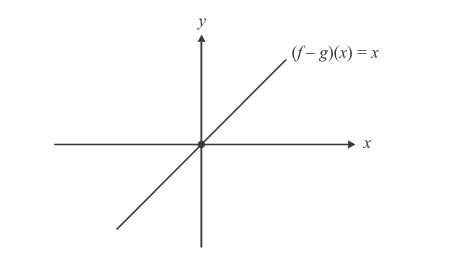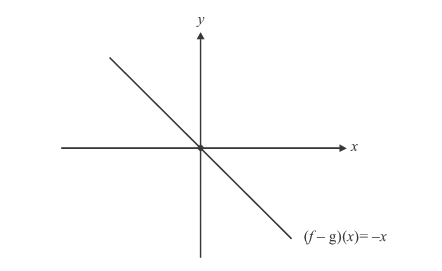# Examples on Functions As Maps

Go back to  'Functions'

Example- 30

Is f(x) = \begin{align}\frac{{x + 1}}{{x + 2}}\end{align} one-one or many-one?

Solution: As described earlier, solve the equation $$f({x_1}) = f({x_2})$$

$$\Rightarrow$$   \begin{align}\frac{{{x_1} + 1}}{{{x_1} + 2}} = \frac{{{x_2} + 1}}{{{x_2} + 2}}\end{align}   $$\Rightarrow$$    \begin{align}{x_1}{x_2} + 2{x_1} + {x_2} + 2 = {x_1}{x_2} + {x_1} + 2{x_2} + 2\end{align}

$$\Rightarrow$$     $${x_1} = {\rm{ }}{x_2}$$

$$\Rightarrow$$     $$\text{The function is one-one.}$$

Example- 31

Is f(x) = \begin{align}\frac{{{x^2} - 8x + 18}}{{{x^2} + 4x + 30}}\end{align} one-one or many one?

Solution: Again solving $$f({x_1}) = f({x_2})$$ gives

\begin{align} &\frac{{x_1^2 - 8{x_1} + 18}}{{x_1^2 + 4{x_1} + 30}} = \frac{{x_2^2 - 8{x_2} + 18}}{{x_2^2 + 4{x_2} + 30}}\\\\&\Rightarrow\ 4x_1^2{x_2} + 30x_1^2 - 8{x_1}x_2^2 - 240{x_1} + 18x_2^2 + 72{x_2}\\\\&= 4{x_1}x_2^2 + 30x_2^2 - 8x_1^2{x_2} - 240{x_2} + 18x_1^2 + 72{x_1}\\\\&\Rightarrow12{x_1}{x_2}\left( {{x_1} - {x_2}} \right) + \,12\left( {{x_1} + {x_2}} \right)\left( {{x_1} - {x_2}} \right)\, - \,312\left( {{x_1} - {x_2}} \right) = 0\\\\&\Rightarrow ({x_1} - {x_2})\{ {x_1}{x_2} + {x_1} + {x_2} - 26\} = 0\end{align}

We see that $$f({x_1}) = f({x_2})$$ does not uniquely imply $${x_1} = {x_2}$$, since the quantity in the braces could also be 0.

Hence, this function is many-one.

Example- 32

Are the following functions into or onto ?

(a)   $$f:\mathbb{R} \to \mathbb{R}$$ $$\qquad\qquad\qquad\qquad f(x) = \sin x$$

(b)   $$f:\left[ { - 1,\,\,1} \right] \to \left[ {0,\,\,1} \right] \qquad\qquad f(x) = {x^2}$$

(c)   $$f:(0,\,\,\infty ) \to \mathbb{R} \qquad\qquad\qquad f(x) = \ln x$$

(d)   $$f:(1,\,\,\infty ) \to \mathbb{R} \qquad\qquad\qquad f(x) = \ln x$$

Solution: (a)  The range for the sin function is [–1, 1]. The co-domain has been specified as $$\mathbb{R}$$.

Hence, $$f$$ is into

(b)  For the specified domain, the range is [0, 1], same as the co-domain.f  is onto.

(c)  For domain (0, $$\infty$$ ),$$\ln x$$ can take on any real value (refer to the section on standard functions).

$$f$$ is onto.

(d)  For the domain (1, $$\infty$$), $$\ln x$$ achieves a minimum at $$x = 1.$$ (ln $$1=0$$)

Hence $$\ln x$$ > 0 for the specified domain, i.e., the range is (0,$$\infty$$). $$f$$ is into

Example– 33

Consider   $$f(x) = \left\{ \begin{array}{l}x\;{\rm{ if }}\;x\;{\rm{\;is\;rational}}\,\,\,\\0\;{\rm{\; if\; }}x{\rm{ \;is\; irrational}}\end{array} \right\}g(x) = \left\{ \begin{array}{l}0\;{\rm{\; if \; }}x\;{\rm{ \;is \;rational}}\,\,\,\\x\;{\rm{\; if \; }}x{\rm{\;is\; irrational}}\end{array} \right\}$$. Is  $$f - g$$ one-one or many-one? Is it onto or into?

Solution:

$(f - g)(x) = \left\{ \begin{gathered} x{\text{ if }}x{\text{ is rational}}\,\,\, \hfill \\ - x{\text{ if }}x{\text{ is irrational}} \hfill \\ \end{gathered} \right\}\,\,\,\,\,\,\left( \begin{gathered} {\text{Here co - domain has not been specified }} \hfill \\ {\text{explicitly, so assume it to be }}\mathbb{R} \hfill \\ \end{gathered} \right)$

A little thinking shows that each input is assigned to only one output.

Hence, $$f - g$$  is one-one

Also, every real number has a corresponding pre-image. For example, the pre-image of  $$\sqrt 2 \;{\rm{ }}\;is{\rm{ - }}\sqrt 2 {\rm{ }},\;{\rm{}}\;of{\rm{ - }}\sqrt 2\; {\rm{ }}is\;{\rm{}}\sqrt 2 {\rm{\; }}of{\rm{\; }}3/5{\rm{ \;}}is{\rm{\; }}3/5$$  and so on.

Hence,  $$f - g$$  is onto

If you are not convinced, think of this in the following way. Suppose you construct an axes with two ‘rational lines’ instead of  two real lines. These two lines contain all (and only) the rational points. What will $$(f-g)(x)$$ look like for this axes?

$$(f-g)(x)=x$$ if $$x$$ is rationalFor the rational axes, $$(f-g)(x)$$ is one-one and onto

Doing this for an irrational axes gives us the following graph.For the irrational axes, $$(f-g)(x)$$ is one-one and onto.

Now superimpose the two axes, and you get the normal real axes. (The rational and irrational lines are exactly complementary. They do not overlap and together they form the real line)

The two parts of $$(f-g)(x)$$ are separately one-one and onto. When you combine them, the resultant is obviously also one-one and onto.

Hence, $$f - g$$ is one-one and onto.

## TRY YOURSELF - IV

Q. 1 How many distinct linear maps, map [–1, 1] onto [0, 2]?

Q. 2 Analyse the following functions for being one-one/many-one and onto/into.

(a) $$f:[0,\infty ) \to \mathbb{R}\,\,\,\,\,\,\,\,\,\,f\left( x \right) = {x^2} + 3x + 2$$

(b) $$f:[0,\infty )\,\, \to \,\,[0,\infty )\,\,\,\,\,\,\,\,f\left( x \right) = \frac{x}{{1 + x}}$$

Q. 3 Let $$n\left( A \right){\rm{ }} = {\rm{ }}4{\rm{ \;}}and{\rm{ \;}}n\left( B \right){\rm{ }} = {\rm{ }}6$$. Find the number of one-one functions from A to B.

Q. 4 Let  $$g(x) = {x^2} - 4x - 5$$. Which of the following statements are true?

(a) g is one-one on $$\mathbb{R}$$

(b) g is one-one on $$( - \infty ,2]$$

(c) g is not one-one on $$( - \infty ,4]$$

(d) g is one-one on   $$( - \infty ,0)$$

Functions
grade 11 | Questions Set 1
Functions
Functions
grade 11 | Questions Set 2
Functions